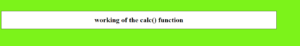# How to Set Calc Viewport Units Workaround in CSS ?

• Last Updated : 30 Jun, 2020

In the HTML webpage, when applying values to the CSS properties, the calculations are performed by using calc() function.

Syntax:

```element {

// CSS property
property : calc( expression)
}
```

Properties: The calc() function takes parameters in the form of a single expression. The value becomes the result of the expression. Even the expression be the combination of many operators follow the standard precedence rules.

• – Subtraction
• * Multiplication. Any of the arguments should be a number
• / Division. The right-hand side should be a number

One must be careful while writing the syntax and must take care of the following points.

1. The + and operator must be surrounded by white space i.e. height(100%-30px) is considered to be invalid but height(100% – 30px) is a valid expression. For the / and * operator, the white spacing is not necessary but is highly recommended.
2. Division by 0 results in an error.

Example 1:

 ```<``html` `lang``=``"en"``>`` ` `<``head``>``    ``<``meta` `charset``=``"UTF-8"``>``    ``<``meta` `name``=``"viewport"` `content``=``        ``"width=device-width, initial-scale=1.0"``>``    ``<``title``>``        ``CSS Calc Viewport Units Workaround``    ````     ` `    ``<``style``>``        ``body {``            ``background-color: rgb(30, 240, 30);``        ``}`` ` `        ``h1 {``            ``color: rgb(30, 240, 30);``        ``}`` ` `        ``div {``            ``width: calc(100% - 100px);`` ` `            ``/* Using the calc() func to change the ``               ``width of the div element by 100px */``            ``border: 1px solid black;``            ``background-color: white;``            ``margin-top: 50px;``            ``text-align: center;``        ``}``    `````` ` `<``body``>``    ``<``div``>``        ``<``h1``>working of the calc() function``    `````` ` ``

output:Example 2:

 ```<``html` `lang``=``"en"``>`` ` `<``head``>``    ``<``meta` `charset``=``"UTF-8"``>``    ``<``meta` `name``=``"viewport"` `content``=``        ``"width=device-width, initial-scale=1.0"``>`` ` `    ``<``title``>``        ``CSS Calc Viewport Units Workaround``    ```` ` `    ``<``style``>``        ``body {``            ``background-color: rgb(30, 240, 30);``        ``}`` ` `        ``h1 {``            ``color: rgb(30, 240, 30);`` ` `            ``/* Using the calc() func to change ``            ``the font size of the h1 element */``            ``font-size: calc(1.5rem + 3vw);``        ``}`` ` `        ``div {``            ``width: calc(100% - 100px);``            ``border: 1px solid black;``            ``background-color: white;``            ``margin-top: 50px;``            ``text-align: center;``        ``}``    `````` ` `<``body``>``    ``<``div``>``        ``<``h1``> working of the calc() function``    `````` ` ``

Output:My Personal Notes arrow_drop_up